# Precalculus : Find the Product of a Vector and a Scalar

## Example Questions

### Example Question #1 : Find The Product Of A Vector And A Scalar

Find the product of the vector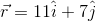and scalar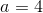.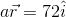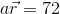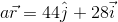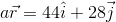Explanation:

When multiplying a vector by a scalar we multiply each component of the vector by the scalar and the result is a vector: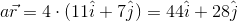### Example Question #2 : Find The Product Of A Vector And A Scalar

Find the vector given by the product: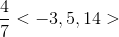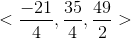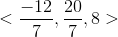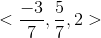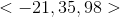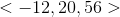Explanation:

Given a scalar k and a vector v, the vector given by their products is defined component-wise: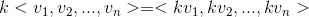.

Here, our product is: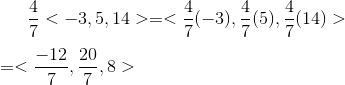### Example Question #3 : Find The Product Of A Vector And A Scalar

This question refers to the previous question.

Simplify.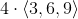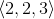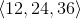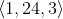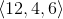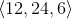Explanation:

In order to simplify this problem we need to multiply the scalar factor to each component of the vector.

In our case the scalar factor isThus,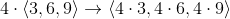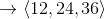### Example Question #4 : Find The Product Of A Vector And A Scalar

Evaluate: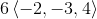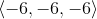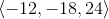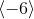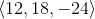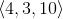Explanation:

In order to determine the final value of the vector, distribute the scalar among each term in the vector.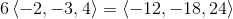### Example Question #5 : Find The Product Of A Vector And A Scalar

If the vector from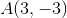to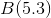was multiplied by a scale factor of 3, what is the new vector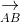?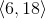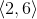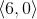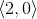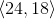Explanation:

To find:

Subtract vectorfrom.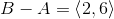Multiply this vector by a scale of 3.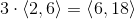### Example Question #6 : Find The Product Of A Vector And A Scalar

Find the product of: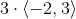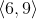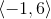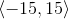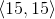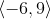Explanation:

When a scalar is multiplied to a vector, simply distribute that value for both terms in the vector.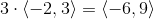### Example Question #7 : Find The Product Of A Vector And A Scalar

When given a vectorand a scalar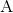what happens to the length and angle ofwhen multiplied with?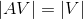or the length of the product is the same as the original vector.

The angle is unchanged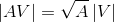or the length of the product is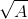times as long as the original vector.

The angle is multiplied by.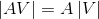or the length of the product istimes the length of the original vector.

The angle is multiplied byor the length of the product is the same as the original vector.

The angle is multiplied by.or the length of the product istimes the length of the original vector.

The angle is unchanged.or the length of the product istimes the length of the original vector.

The angle is unchanged.

Explanation:

In simple termsis the hypotenuse of a triangle formed by the components of. So when you multiplybyit mupltiplies all the componets by. This makes the length of the hypotensuse grow byas demonstrated by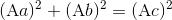from the Pythagorean Theorem.

For the same reasons the angle does not change because the new longer triangle will be a similar triangle to the original triangle.

### Example Question #8 : Find The Product Of A Vector And A Scalar

Determine the product: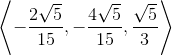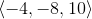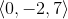Explanation:

To find the product of the scalar and the vector, simply multiply the scalar throughout each term inside the vector.  Do not confuse this with the dot product or the norm of a vector.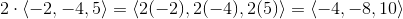The answer is:### All Precalculus Resources